Sergey Horban is a QA Engineer with 3+ years of experience in automation and manual testing, bug-tracking and test management.

Become a JMeter and Continuous Testing Pro

Start Learning

## Test Your Website Performance NOW!Please enter a URL with http(s)
Feb 19 2018

# How to Work With Strings in JMeter

In this article, I will present the most common and frequently used methods of working with strings when writing tests in Apache JMeter™. To learn how to create strings in JMeter, you can look at this blog post that I wrote about it.

# Printing Messages to the Console Using String Concatenation

The output of messages to the console is a pretty good practice for debugging autotests. When writing autotests for APIs that have extensive logic and with numerous checks of the database, it is impossible to debug and track the execution status of each step of the test case without showing messages in the console. These messages help quickly determine at what stage of the test operation a failure occurred or a bug was detected.

When outputting messages to the console, we often use string concatenation. A string concatenation is the union of several strings into one. In Java, the concatenation of multiple strings is performed in different ways, which differ in their performance. One option is to use the "+" operator. In the blog post “How to Initialize Database Variables and Assign Them Values in JMeter”, this method is often found in code examples.

In order to perform string concatenation, you need to do the following:

3. JSR223 Sampler -> Language Java (BeanShell 2.0b5/BeanShell Engine 1.0) You can also choose Groovy.

Java (BeanShell 2.0b5 / BeanShell Engine 1.0) allows you to use the Java language syntax in JMeter.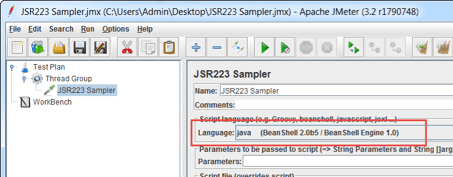4. Open the JMeter Console. The JMeter console will be used to display the output of the code.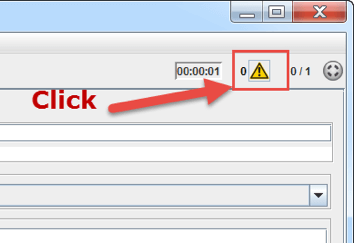5. In the JSR223 Sampler, add the following code example.

```setStrictJava (true);

String a = "Jmeter";
String b = "3.2";

log.info(a + b);
log.info("!!!" + a + b + "!!!!");
log.info("Jmeter" + "3.2");

String s = "Jmeter" + "3.2";
log.info(s);
```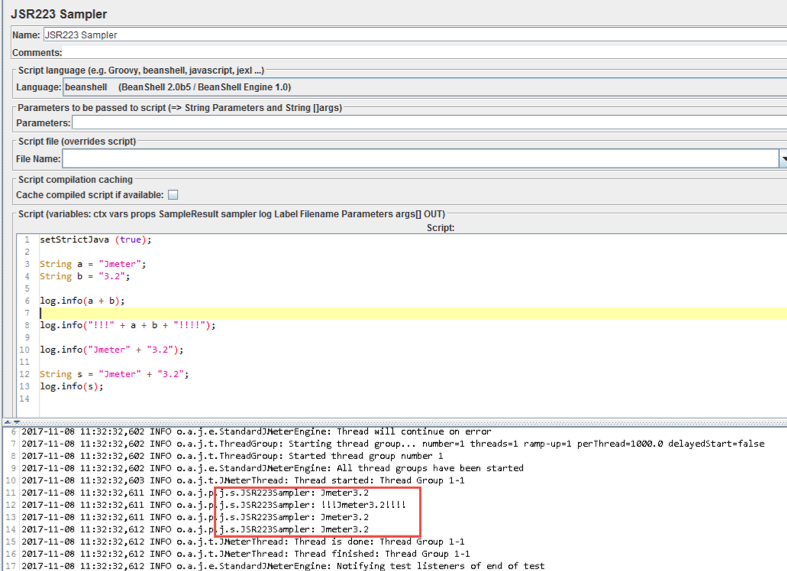Let's analyze in more detail what this code does:

• String a = "Jmeter" and String b = "3.2" - declaring variables and assigning them values
• a + b is the operation of concatenating a string value of one variable with a string value of the second variable
• "!!!" + a + b + "!!!!" - concatenation of string literals with the values of string variables
• log.info () is a method that prints the value to the JMeter console

You can perform string concatenation not only for string literals and variables of String data type, but also for numeric data types.

Example code

```setStrictJava (true);

String a = "Jmeter";
int b = 3;

log.info(a + " " + b);
log.info("!!!" + a + " " + b + "!!!!");
log.info("Jmeter" + " " + 3);

String s = "Jmeter" + " "+ 3;
log.info(s);
```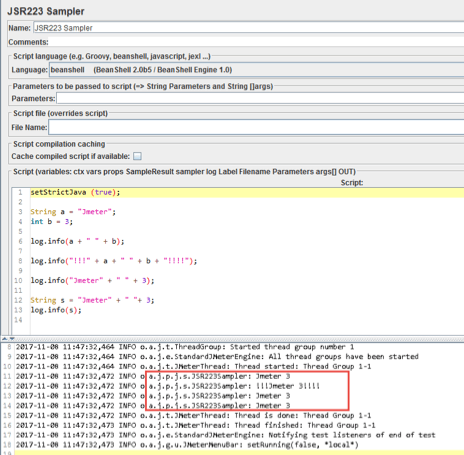This code example shows the concatenation of strings with a numeric data type, resulting in a string.

Another method of concatenating strings is the concat (String str) method, which is more efficient than using the "+" operator.

Example code

```setStrictJava (true);

String a = "Jmeter";
String b = "3.2";

String c = a.concat(b);
log.info(c);

String c1 = "Jme".concat("ter");
log.info(c1);

String c2 = "Jmeter".concat(b);
log.info(c1);
```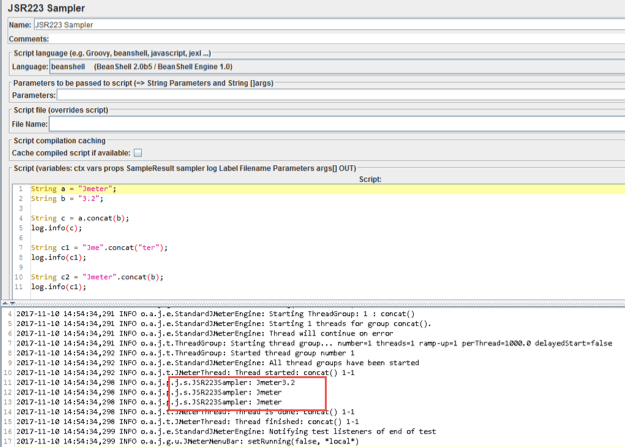The concat (String str) method takes as input only a string literal or a variable with the data type String. Using this method with numeric data types causes an error. Calling this method for a numeric data type also results in an error.

Example code

```int a = 3;
String b = "Jmeter";

String c = a.concat(b);
log.info(c);
```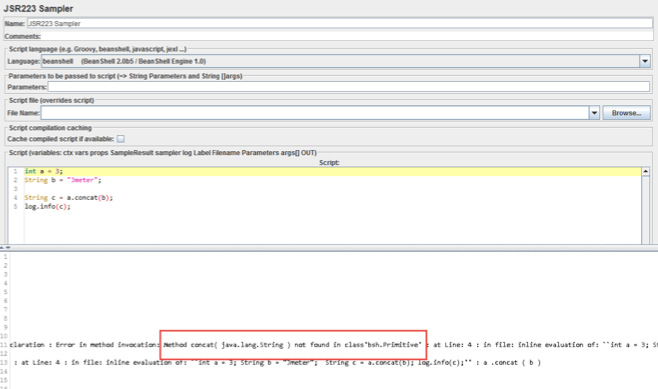The image above shows an example of an error that occurs when the concat (String str) method is called for a numeric data type.

Example code

```int a = 3;
String b = "Jmeter";

String c = b.concat(a);
log.info(c);
```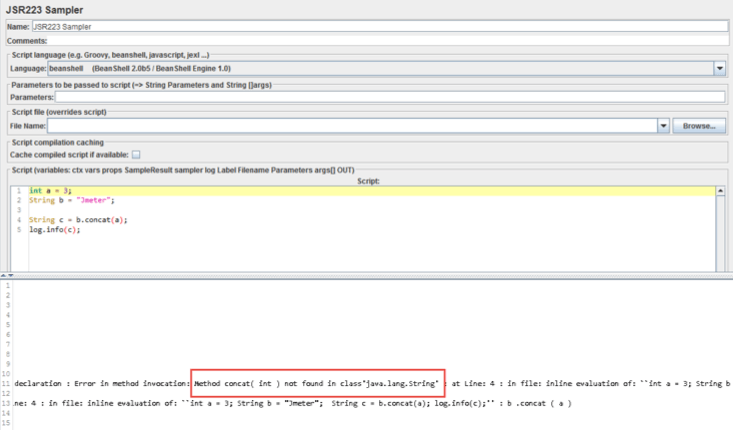The image above shows an example of an error that occurs when a variable with a numeric data type is passed to the concat (String str) method.

# Converting Different Data Types to a String Using the toString () Method

This method is used to convert primitive data types to a string. This method also allows you to convert values that are contained in an object to a string. To make the toString () method convert values that are contained in an object to a string, this method must be implemented in the class on which the object was created.

Example code

```setStrictJava (true);

setStrictJava (true);

int a = 1;

log.info(a.toString());
```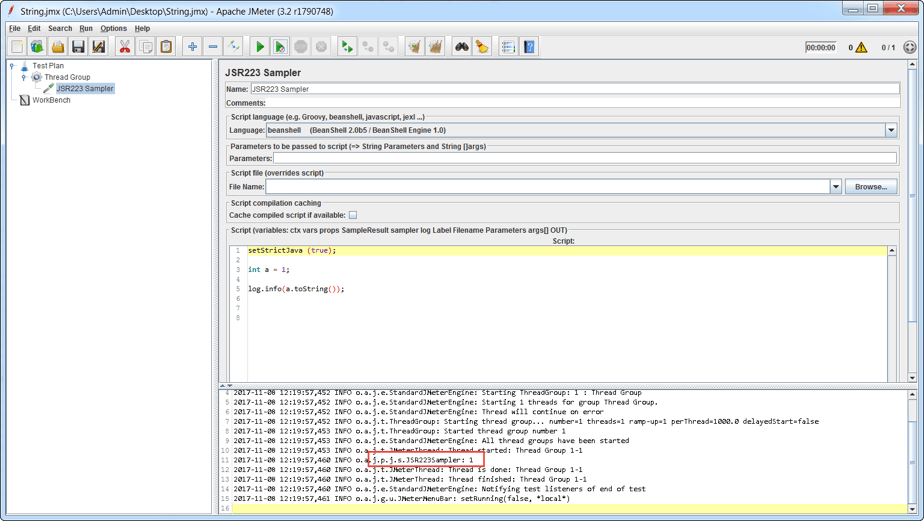This code example shows the conversion of a variable value of an int data type into a string.

It’s recommended to convert object values to a string based on the ArrayList. The ArrayList is a data structure that is indexed, dynamically expanding, and shrinking.

Example code

```setStrictJava (true);

ArrayList arr = new ArrayList();
log.info(arr.toString());
```

• ArrayList arr = new ArrayList () - creates an object with a raw data type ArrayList
• log.info (arr.toString ()) - the first arr.toString () converts the values of the object into a string, and then log.info () prints this value to the JMeter console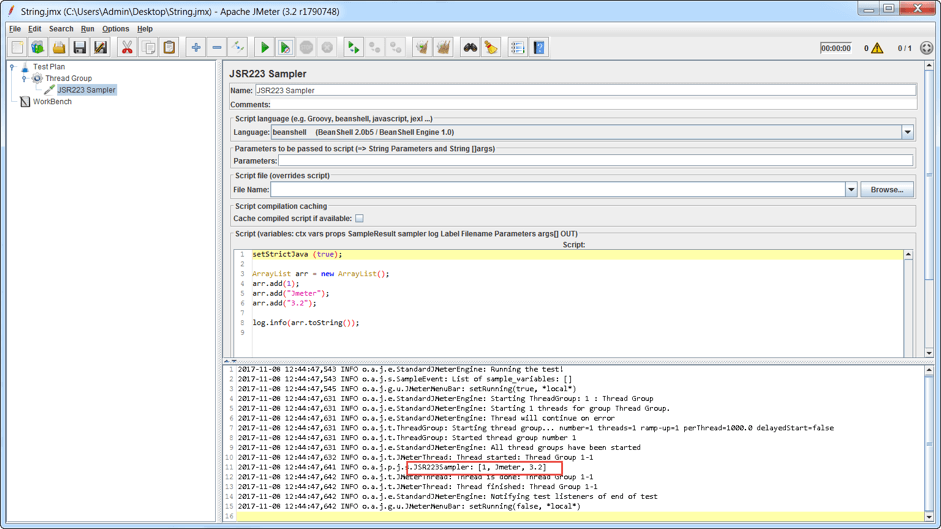If the toString () method is not implemented in the class on which the object was created, the method simply returns the value of the reference to this object.

Example code

<

```setStrictJava (true);

int [] arr = {1,2,3,4};

log.info(arr.toString());
```

• int [] arr = {1,2,3,4} - creates an array that can only contain values of data type int. An array is a data structure that stores values of the same type.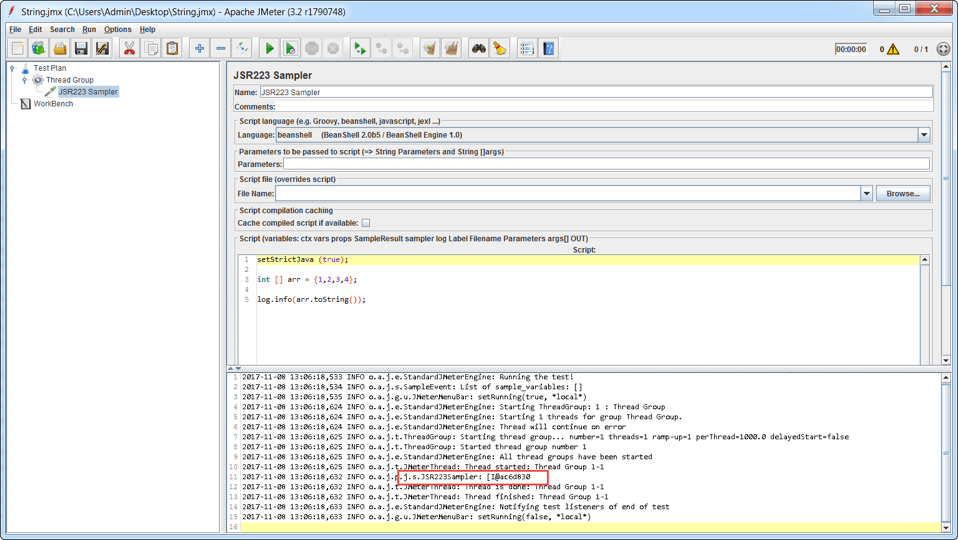In the image above, you can see that the values 1,2,3,4 are not displayed in the console, and that the value of the object reference is displayed.

# Retrieving Data from the API Response Using the Substring () Method

This method allows you to extract a substring from a string. In testing practice, this method is used when the API returns a response and you need to get a specific value from the answer and continue working with the value.

Imagine that we have an API that checks whether the customer has a certain discount for the purchase of goods. If the discount exists, then the answer is returned {"message": "For client a discount of 20%"}. We will assume that the response text never changes, except for the discount value.

In order to get the discount value from the API response, you need to do the following:

The Dummy Sampler is used to simulate a request and response. In our case, the Dummy Sampler will be used to simulate only the response from our prospective API.

2. Go to the archive that you downloaded and copy the jmeter-plugins-dummy-0.2.jar file to the JMeter ... \lib\ext directory.

Since we only need a response for the simulation, the "Response Date" section needs to insert our alleged response. In our case, this is {"message": "For client a discount of 20%"}.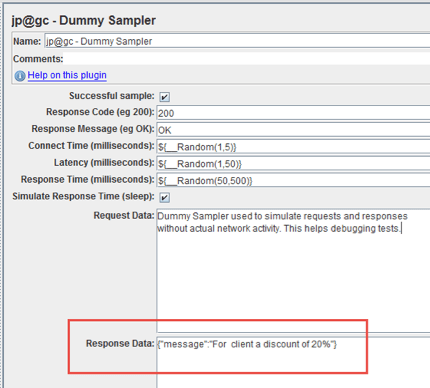5. jp@gc - Dummy Sampler -> Add -> Assertions -> BeanShell Assertion

The "BeanShell Assertion" is the place to add the Java code.

6. Open the JMeter console

The console will be used to display the results of the work of the code.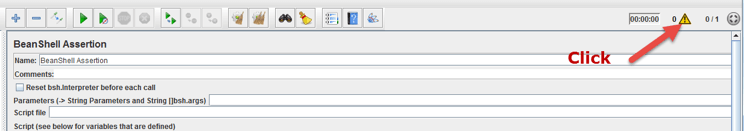7. In the "BeanShell Assertion", add the following code:

Example code

```setStrictJava (true);

String discount = SampleResult.getResponseDataAsString().substring(37,39);

log.info(discount);
```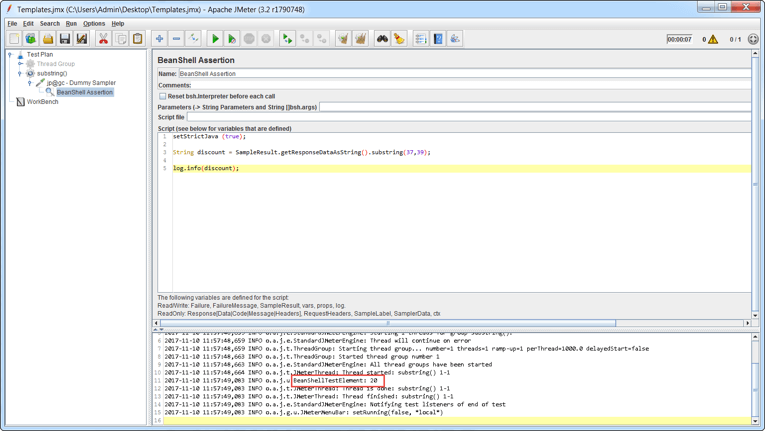Let's analyze in more detail what the code above does:

• String discount - Declaring a variable of data type String
• SampleResult - The name of the class in which the method is implemented getResponseDataAsString ()
• getResponseDataAsString () is a method that returns the result of the work for any "Sampler" element (For example, JDBC Request, HTTP Request, etc.), automatically converting to a string (value of data type String). In our case, the result of working for jp@gc - Dummy Sampler is the value {"message": "For client a discount of 20%"}.
• substring (37,39) - cuts out the value returned by getResponseDataAsString (), substring from position 37 (inclusive) and position 39 (not inclusive). As we already know from the article "Creating Strings in JMeter”, the String is a sequence of characters in Unicode. Based on this, our string {"message": "For client a discount of 20%"} contains 42 characters (When specifying the incoming parameters in the method, it is necessary to take into account that the indexing for the characters in the line begins with 0). When we specify 37,39 in the method, we cut the character from position 37 (in our case this is the number "2") at position 38 (in our case this is the digit "0"). The symbol at position 39 is not taken into account. After the method substring (37,39) cut out the value (in our case it is 20), the "=" operator assigns this value to the variable discount.
• log.info (discount) - displays the variable discount in the JMeter console

The substring () method has a second implementation that allows you to cut a substring from a string, from the specified position to the end of the source string. For example, if we need to cut out the entire message, just specify the index from which to start cutting.

Example code

```setStrictJava (true);

String discount = SampleResult.getResponseDataAsString().substring(10);

log.info(discount);
```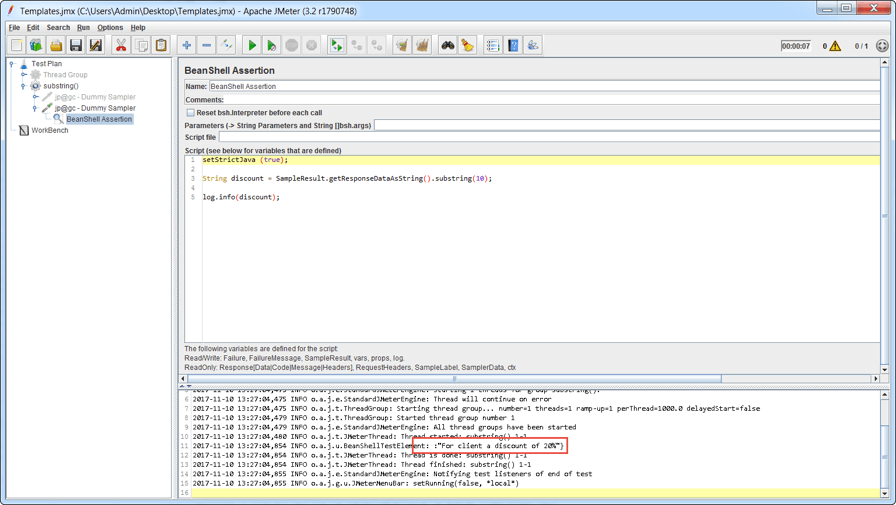In the image above, the substring () method cut the substring from the main string, from the specified index and to the end of the line.

# Comparing Character Strings Using the equals (), equalsIgnoreCase () Method and the "==" operation

Character strings comparison is a very common procedure for creating tests in JMeter. Different methods can be used to compare strings, each with their own uniqueness.

In the section "Retrieving Data from the API Response Using the Substring () Method” in this blog post, we showed an example of a discount value for the client. Now let’s imagine that the API returns various messages and our task is to check that the messages are returned correctly. For example, that the words in the message are not missing, the API returns the required message, etc..

To verify that the API returns the correct message, do the following:

Run steps 1-7 of the section "Retrieving data from the API response using the substring () method"

In the "BeanShell Assertion", add the following code:

Example code

```setStrictJava (true);

String message = "{\"message\":\"For client a discount of 20%\"}";

String messageFromApi = SampleResult.getResponseDataAsString().substring(0);

if(message.equals(messageFromApi)) {

log.info("The message is correct");

} else {

log.info("API returned an incorrect message");
}
```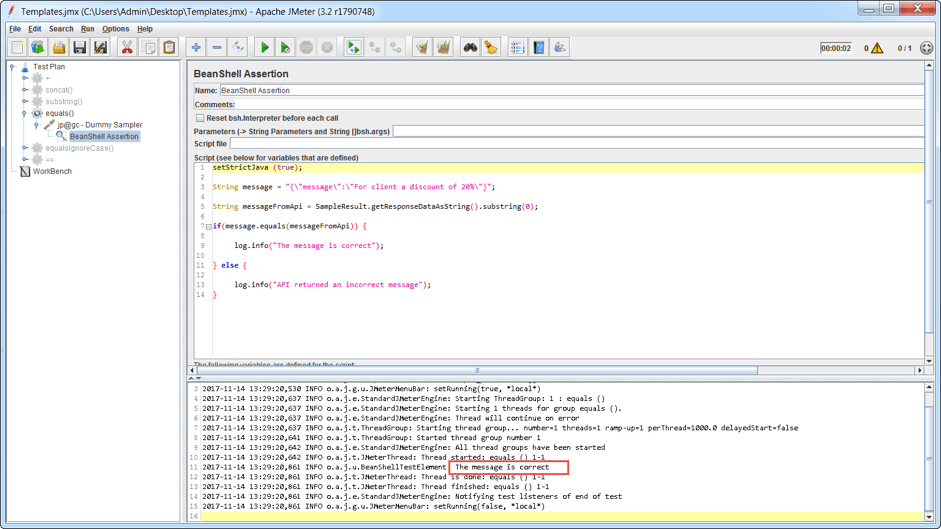In the code example above, the following is described:

• String message = "{\"message\": \"For client a discount of 20%\"}"; - Creates a variable with data type String (string). The value of this variable is our expected message from the API.
• String messageFromApi = SampleResult.getResponseDataAsString().substring (0); - described in detail in "Retrieving data from the API response using the substring () method".
• message.equals (messageFromApi) - the string comparison. In this case, the value of the variable “message” (our expected result) is compared with the value of the variable “messageFromApi” (our actual result, returned from the API). If the compared strings contain the same characters in the same order, the equals () method returns the logical value "true", and otherwise "false". The equals () method compares strings to case sensitivity.
• if else - conditional branch statements. Used to direct the execution of the program in two different branches. Since in our example the compared strings are identical, the equals () method returns a logical value of "true" and the code that is in the block for the “if” statement is executed (in our case this is log.info ("The message is correct"); If the compared strings are not identical, then the code that is in the block for the “else” statement will be executed.

If you need to perform string comparisons, without taking into consideration case sensitivity, then the equalsIgnoreCase () method is used. For this method, the symbols A-Z and a-z are assumed to be the same.

Example code

```setStrictJava (true);

String message = "{\"MeSSage\":\"FOR client A discount OF 20%\"}";

String messageFromApi = SampleResult.getResponseDataAsString().substring(0);

if(message.equalsIgnoreCase(messageFromApi)) {

log.info("The message is correct");

} else {

log.info("API returned an incorrect message");
}
```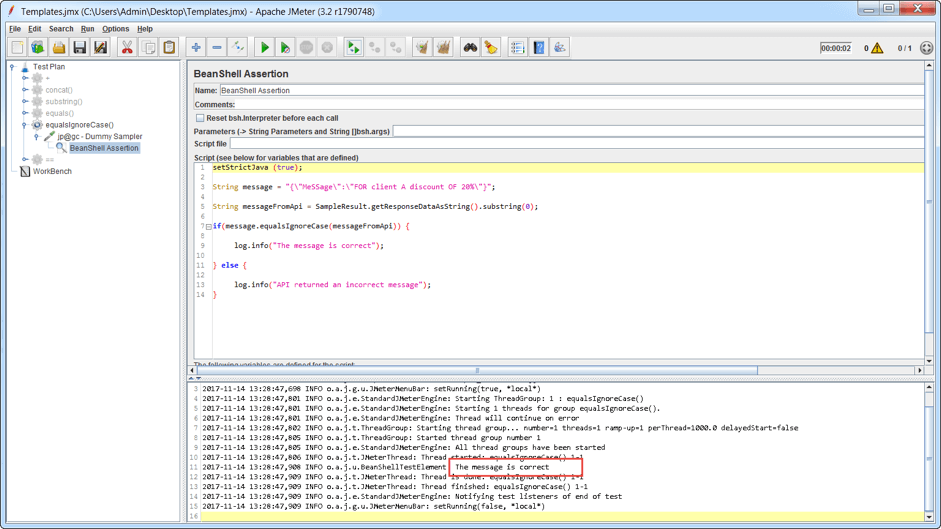The image above shows that the expected message from the API (in our case this is the value of the "message" variable) contains uppercase characters that are not in the actual message (in our case this is the value of the "messageFromApi" variable), but the strings are identical.

For string comparisons, the == operation is sometimes used. As we already know, when you create a reference variable with a String data type, an object with the corresponding value is created in the JVM memory. When comparing two variables with a reference data type using the == operation, a comparison is made to ensure that the two variables refer to the same object in the JVM memory.

The difference between the equals () method and the == operation is shown below.

Example code

```setStrictJava (true);

String message = "{\"message\":\"For client a discount of 20%\"}";

String messageFromApi = SampleResult.getResponseDataAsString().substring(0);

if(message == messageFromApi) {

log.info("The message is correct");

} else {

log.info("API returned an incorrect message");
}

if (message.equals(messageFromApi)) {

log.info("The message is correct");
}
```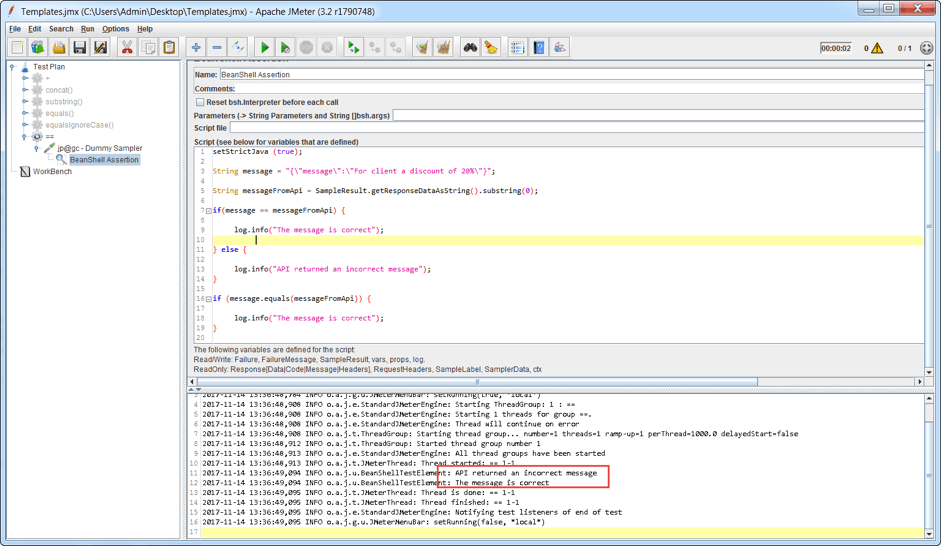The image above shows that the strings are not identical when using the == operation, although the number and sequence of characters are identical. This indicates that the == operation does not compare the values that are stored in the object, but compares the references to the object.

Why is this?

• "==" compares object references
• "equals" compares object values

When we used the method "substring" and created the variable "String messageFromApi", we created a new object in the new memory location, but the value itself did not change. The "String message" is a separate object that has its own memory location. When comparing the variables, "==" looks at the memory cells. If the compared variables have different memory cells, then these variables are not identical.Please enter a URL with http(s)